# How to Add the Current Month or Year in a Cell in Excel

This post will guide you how to add the current month or year into a cell or header or footer in your worksheet. How do I insert the current date or time in a cell with a formula in Excel.

## Add Current Month or Year Using Short Keys

Assuming that you want to insert current year or month into a cell or header in Excel, you can use one short cut to achieve the result. Just do the following steps:

#1 you can press Ctrl + ; keys to insert the current date in a cell.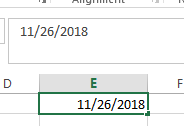#2 press one space to insert one blank character, and then press Ctrl + Shift +; to insert the current time in that cell.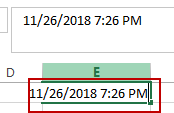## Add Current Month or Year Using Formula

You can also use a formula to insert the current year, month, day or a date or time in one cell.

Insert current Year

To insert the current year into a cell or footer or header in Excel, you can use a formula based on the YEAR function and the TODAY function. Just like this:

`=YEAR(TODAY())`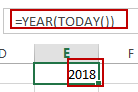Type this formula into a blank cell or footer or header in your worksheet, and then press Enter key in your keyboard.

Insert Current Month

If you want to insert the current month, you can use a formula based on the MONTH function and the TODAY function to achieve the result. Like this:

`=MONTH(TODAY())`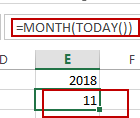Insert Current Day

To Insert the current day in a cell, you can use the below formula based on the TODAY function.

`=TODAY()`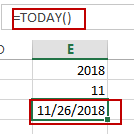Insert Current Date and Time

If you want to insert the current date and time into one cell, you can directly use the NOW function. Like this:

`=NOW()`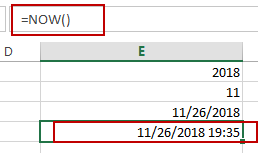### Related Functions

• Excel YEAR function
The Excel YEAR function returns a four-digit year from a given date value, the year is returned as an integer ranging from 1900 to 9999. The syntax of the YEAR function is as below:=YEAR (serial_number)…
• Excel MONTH function
The Excel MONTH function returns the month of a date represented by a serial number. And the month is an integer number from 1 to 12. The syntax of the MONTH function is as below:=MONTH (serial_number)…
• Excel TODAY function
The Excel TODAY function returns the serial number of the current date. So you can get the current system date from the TODAY function. The syntax of the TODAY function is as below:=TODAY()…
• Excel NOW function
The Excel NOW function returns the serial number of the current date and time. And it will be updated each time when your worksheet is changed or opened.The syntax of the NOW function is as below:=now()…
Related Posts

Calculating Average Of The Numbers

Are you weary of investing a lot of time and effort in manually calculating the average of the numbers by including or excluding 0 and calculating the average of the top 3 scores? Then congratulations because you have just landed ...

Add Months To Date In Excel

It is important to adjust time periods when performing financial modeling. In Excel, you can use the MONTH function to add a specific number of months to a date. The MONTH function takes one argument: the number of months you ...

Add Days to Date in MS Excel

If you are an vavid MS Excel user, then you might have come across situations where you need to add the same or different days into the particular date, and if you have done this task manually, then let me ...

Filter Data By Date Field

The use of Microsoft Excel's Format Cells and Auto Filter option is a great way to filter data by year or Month. This article will discuss how to filter by date field using Filter function and Format cells in Microsoft ...

How to Count Dates of Given Year in Excel

This post will guide you how to count Dates of a certain year in the range of dates using a formula in Excel 2013/2016 or Excel office 365. How do I count dates by a given year in Excel. And ...

How to Sum Values Based on Month and Year in Excel

We often do some summary or statistic at the end of one month or one year. In these summary tables, there are at least two columns, one column records the date, and the other column records the sales or product ...

How to Convert Date & Time Format to Date in Excel

Sometimes we want to convert date and time format to date only format in excel for example convert 01/29/2019 06:51:03 to 01/29/2019, we can convert format by Formula or Format Settings. The two ways are easy to learn, so you ...

How to Extract Year from Date & Time Format in Excel

Sometimes we want to get Year information from date and time format to show the Year only in excel. For example convert 01/29/2019 06:51:03 to 2019, we can get Year information by Formula or Format Settings. The two ways are ...

How to Calculate Remaining Days in a Month or Year in Excel

This post will guide you how to calculate remaining days in a given month or year in Excel. How do I calculate the number of days left in a month or year using a formula in Excel 2013/2016. Calculate Remaining ...

How to Split Date into Day, Month and Year in Excel

This post will guide you how to split date into separate day, month and year in excel. How do I quickly split date as Day, Month and Year using Formulas or Text to Columns feature in Excel. Split Date into ...

Sidebar